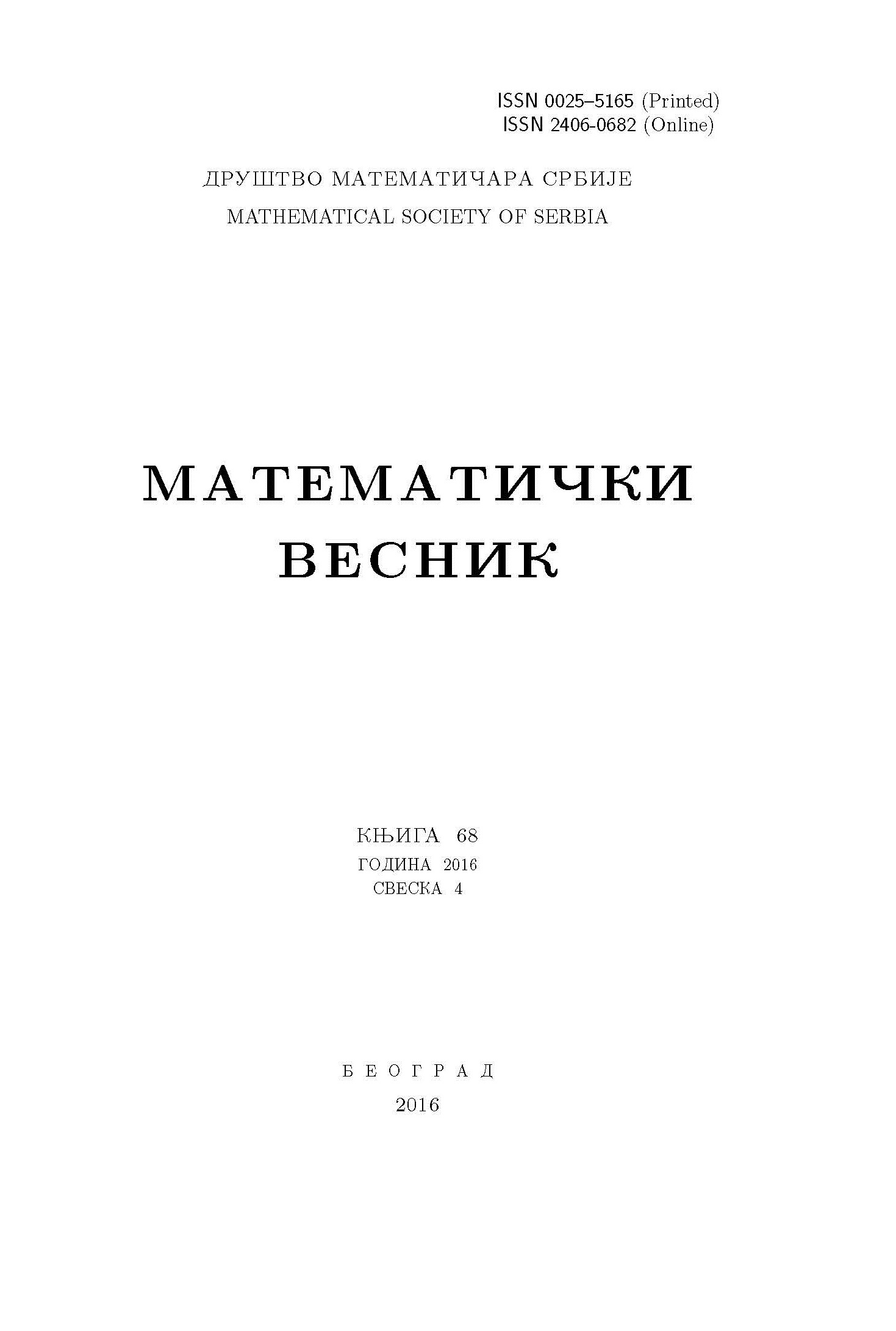﻿ Matematički Vesnik ﻿
 MATEMATIČKI VESNIK МАТЕМАТИЧКИ ВЕСНИКEQUIPRIME FUZZY GRAPH OF A NEARRING WITH RESPECT TO A LEVEL IDEAL B. Jagadeesha, B. S. Kedukodi, S. P. Kuncham AbstractIn this paper, we introduce an equiprime fuzzy graph of a nearring with respect to the level ideal of a fuzzy ideal. We interrelate graph theoretical properties of the graph and ideal theoretical properties of nearring. We show that the properties like vertex cut, connectedness of the graph depend on the properties of the fuzzy ideal. We define ideal symmetry of the graph and find conditions for the graph to be ideal symmetric. If the fuzzy ideal is equiprime then we show that the level set induces a fuzzy clique. We find conditions required for the level set to be the vertex cover of the graph. We find interrelation between equiprime fuzzy graph and fuzzy graph of nearring with respect to level ideal. We study properties of the graph under neaaring homomorphism. We prove that the connectedness of the graph in homomorphic image depends on properties of ideal. We obtain conditions required for homomorphic image of an equiprime fuzzy ideal to be an equiprime fuzzy ideal.Keywords: Nearring; equiprime; ideal; fuzzy graph; homomorphism. MSC: 16Y30, 03E72, 16Y99 Pages:  1$-$18

﻿